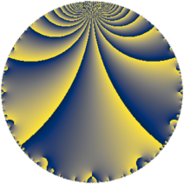# Properties

 Label 147.4.cLevel $147$ Weight $4$ Character orbit 147.c Rep. character $\chi_{147}(146,\cdot)$ Character field $\Q$ Dimension $36$ Newform subspaces $2$ Sturm bound $74$ Trace bound $1$

# Related objects

## Defining parameters

 Level: $$N$$ $$=$$ $$147 = 3 \cdot 7^{2}$$ Weight: $$k$$ $$=$$ $$4$$ Character orbit: $$[\chi]$$ $$=$$ 147.c (of order $$2$$ and degree $$1$$) Character conductor: $$\operatorname{cond}(\chi)$$ $$=$$ $$21$$ Character field: $$\Q$$ Newform subspaces: $$2$$ Sturm bound: $$74$$ Trace bound: $$1$$ Distinguishing $$T_p$$: $$2$$

## Dimensions

The following table gives the dimensions of various subspaces of $$M_{4}(147, [\chi])$$.

Total New Old
Modular forms 64 44 20
Cusp forms 48 36 12
Eisenstein series 16 8 8

## Trace form

 $$36 q - 124 q^{4} - 58 q^{9} + O(q^{10})$$ $$36 q - 124 q^{4} - 58 q^{9} + 262 q^{15} + 596 q^{16} - 164 q^{18} - 652 q^{22} + 828 q^{25} - 60 q^{30} + 1436 q^{36} - 1096 q^{37} - 1516 q^{39} - 1636 q^{43} + 2528 q^{46} + 522 q^{51} + 2302 q^{57} + 644 q^{58} - 3996 q^{60} - 4052 q^{64} + 2208 q^{67} - 2048 q^{72} + 6544 q^{78} - 564 q^{79} - 3642 q^{81} - 3396 q^{85} + 484 q^{88} - 274 q^{93} - 2034 q^{99} + O(q^{100})$$

## Decomposition of $$S_{4}^{\mathrm{new}}(147, [\chi])$$ into newform subspaces

Label Dim $A$ Field CM Traces $q$-expansion
$a_{2}$ $a_{3}$ $a_{5}$ $a_{7}$
147.4.c.a $12$ $8.673$ $$\mathbb{Q}[x]/(x^{12} - \cdots)$$ None $$0$$ $$0$$ $$0$$ $$0$$ $$q+\beta _{1}q^{2}+\beta _{9}q^{3}+(-2+\beta _{11})q^{4}+\cdots$$
147.4.c.b $24$ $8.673$ None $$0$$ $$0$$ $$0$$ $$0$$

## Decomposition of $$S_{4}^{\mathrm{old}}(147, [\chi])$$ into lower level spaces

$$S_{4}^{\mathrm{old}}(147, [\chi]) \cong$$ $$S_{4}^{\mathrm{new}}(21, [\chi])$$$$^{\oplus 2}$$